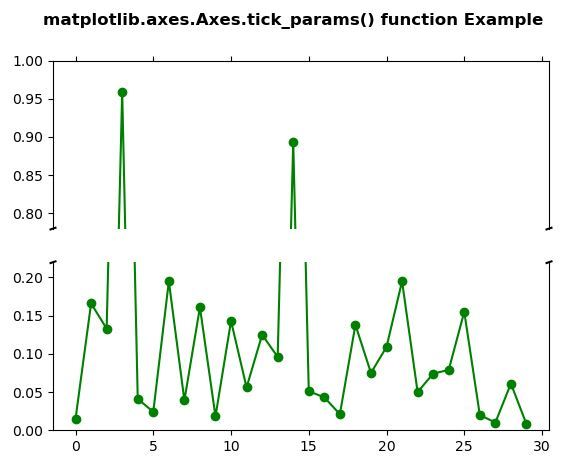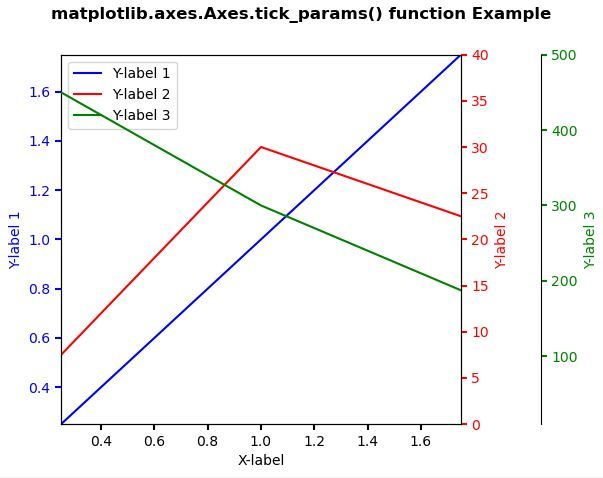Related Articles
Matplotlib.axes.Axes.tick_params() in Python
• Last Updated : 21 Apr, 2020

Matplotlib is a library in Python and it is numerical – mathematical extension for NumPy library. The Axes Class contains most of the figure elements: Axis, Tick, Line2D, Text, Polygon, etc., and sets the coordinate system. And the instances of Axes supports callbacks through a callbacks attribute.

## matplotlib.axes.Axes.tick_params() Function

The Axes.tick_params() function in axes module of matplotlib library is used to control behavior of major tick locators.

Syntax: Axes.tick_params(self, axis=’both’, **kwargs)

Parameters: This method accepts the following parameters.

• axis : This parameter is the used to which axis to apply the parameters to.

Return value: This method does not returns any value.

Below examples illustrate the matplotlib.axes.Axes.tick_params() function in matplotlib.axes:

Example 1:

 `# Implementation of matplotlib function``import` `matplotlib.pyplot as plt``import` `numpy as np`` ` `values ``=` `np.array([``    ``0.015``, ``0.166``, ``0.133``, ``0.159``, ``0.041``,``    ``0.024``, ``0.195``, ``0.039``, ``0.161``, ``0.018``,``    ``0.143``, ``0.056``, ``0.125``, ``0.096``, ``0.094``,``    ``0.051``, ``0.043``, ``0.021``, ``0.138``, ``0.075``,``    ``0.109``, ``0.195``, ``0.050``, ``0.074``, ``0.079``,``    ``0.155``, ``0.020``, ``0.010``, ``0.061``, ``0.008``])`` ` `values[[``3``, ``14``]] ``+``=` `.``8`` ` `fig, (ax, ax2) ``=` `plt.subplots(``2``, ``1``, sharex ``=` `True``)`` ` `ax.plot(values, ``"o-"``, color ``=``"green"``)``ax2.plot(values, ``"o-"``, color ``=``"green"``)`` ` `ax.set_ylim(.``78``, ``1.``) ``ax2.set_ylim(``0``, .``22``)`` ` `ax.spines[``'bottom'``].set_visible(``False``)``ax2.spines[``'top'``].set_visible(``False``)``ax.xaxis.tick_top()``ax.tick_params(labeltop ``=` `False``)``ax2.xaxis.tick_bottom()`` ` `d ``=` `.``005``kwargs ``=` `dict``(transform ``=` `ax.transAxes,``              ``color ``=``'k'``, clip_on ``=` `False``)`` ` `ax.plot((``-``d, ``+``d), (``-``d, ``+``d), ``*``*``kwargs)       ``ax.plot((``1` `-` `d, ``1` `+` `d), (``-``d, ``+``d), ``*``*``kwargs) `` ` `kwargs.update(transform ``=` `ax2.transAxes)  ``ax2.plot((``-``d, ``+``d), (``1` `-` `d, ``1` `+` `d), ``*``*``kwargs)``ax2.plot((``1` `-` `d, ``1` `+` `d), (``1` `-` `d, ``1` `+` `d), ``*``*``kwargs) `` ` `fig.suptitle('matplotlib.axes.Axes.tick_params()\``function Example\n\n', fontweight ``=``"bold"``)`` ` `plt.show()`

Output:Example 2:

 `# Implementation of matplotlib function``import` `matplotlib.pyplot as plt`` ` ` ` `def` `make_patch_spines_invisible(ax):``    ``ax.set_frame_on(``True``)``    ``ax.patch.set_visible(``False``)``    ``for` `sp ``in` `ax.spines.values():``        ``sp.set_visible(``False``)`` ` ` ` `fig, host ``=` `plt.subplots()``fig.subplots_adjust(right ``=` `0.75``)`` ` `par1 ``=` `host.twinx()``par2 ``=` `host.twinx()`` ` `# Offset the right spine of par2.``# The ticks and label have already been``# placed on the right by twinx above.``par2.spines[``"right"``].set_position((``"axes"``, ``                                   ``1.2``))`` ` `# Having been created by twinx, par2 ``# has its frame off, so the line of its``# detached spine is invisible.  First,``# activate the frame but make the patch``# and spines invisible.``make_patch_spines_invisible(par2)`` ` `# Second, show the right spine.``par2.spines[``"right"``].set_visible(``True``)`` ` `p1, ``=` `host.plot([``0``, ``1``, ``2``], [``0``, ``1``, ``2``], ``"b-"``,``                ``label ``=``"Y-label 1"``)`` ` `p2, ``=` `par1.plot([``0``, ``1``, ``2``], [``0``, ``30``, ``20``], ``                ``"r-"``, label ``=``"Y-label 2"``)`` ` `p3, ``=` `par2.plot([``0``, ``1``, ``2``], [``500``, ``300``, ``150``], ``                ``"g-"``, label ``=``"Y-label 3"``)`` ` `host.set_xlim(``0.25``, ``1.75``)``host.set_ylim(``0.25``, ``1.75``)``par1.set_ylim(``0``, ``40``)``par2.set_ylim(``10``, ``500``)`` ` `host.set_xlabel(``"X-label"``)``host.set_ylabel(``"Y-label 1"``)``par1.set_ylabel(``"Y-label 2"``)``par2.set_ylabel(``"Y-label 3"``)`` ` `host.yaxis.label.set_color(p1.get_color())``par1.yaxis.label.set_color(p2.get_color())``par2.yaxis.label.set_color(p3.get_color())`` ` `tkw ``=` `dict``(size ``=` `4``, width ``=` `1.5``)``host.tick_params(axis ``=``'y'``,``                 ``colors ``=` `p1.get_color(),``                 ``*``*``tkw)`` ` `par1.tick_params(axis ``=``'y'``,``                 ``colors ``=` `p2.get_color(),``                 ``*``*``tkw)`` ` `par2.tick_params(axis ``=``'y'``,``                 ``colors ``=` `p3.get_color(),``                 ``*``*``tkw)`` ` `host.tick_params(axis ``=``'x'``, ``*``*``tkw)`` ` `lines ``=` `[p1, p2, p3]`` ` `host.legend(lines, [l.get_label() ``for` `l ``in` `lines])`` ` ` ` `fig.suptitle('matplotlib.axes.Axes.tick_params()\``function Example\n\n', fontweight ``=``"bold"``)`` ` `plt.show()`

Output:Attention geek! Strengthen your foundations with the Python Programming Foundation Course and learn the basics.

To begin with, your interview preparations Enhance your Data Structures concepts with the Python DS Course. And to begin with your Machine Learning Journey, join the Machine Learning – Basic Level Course

My Personal Notes arrow_drop_up